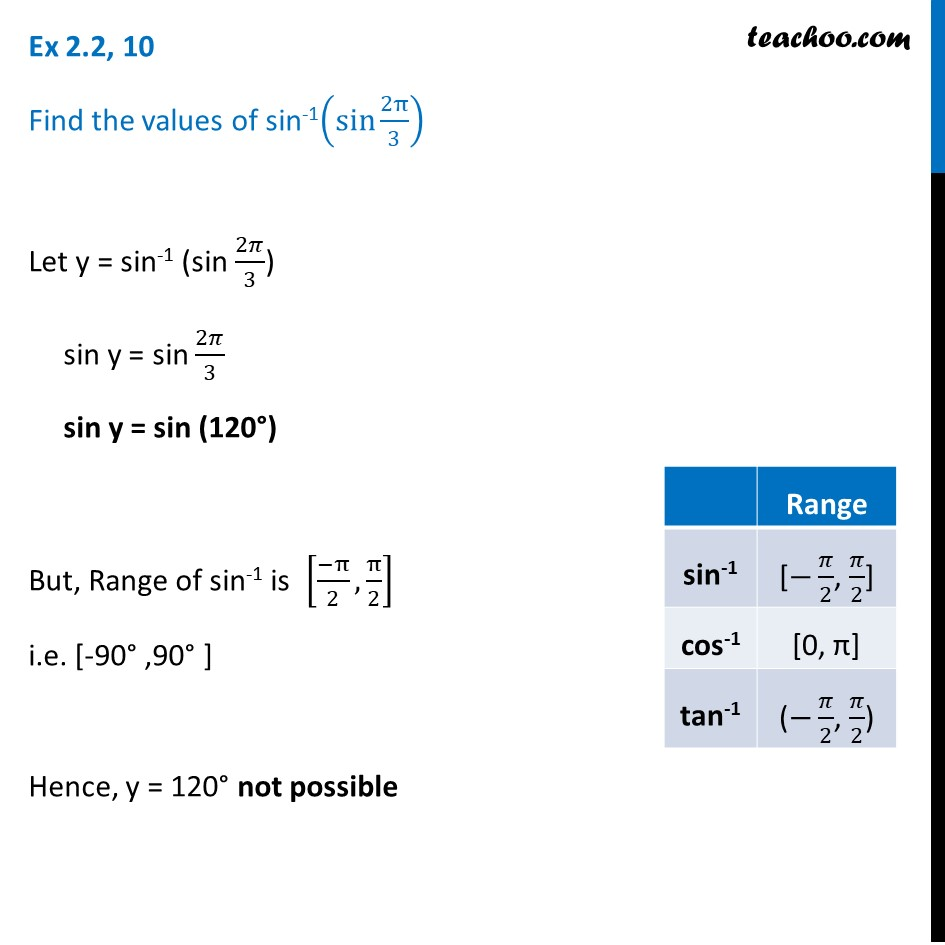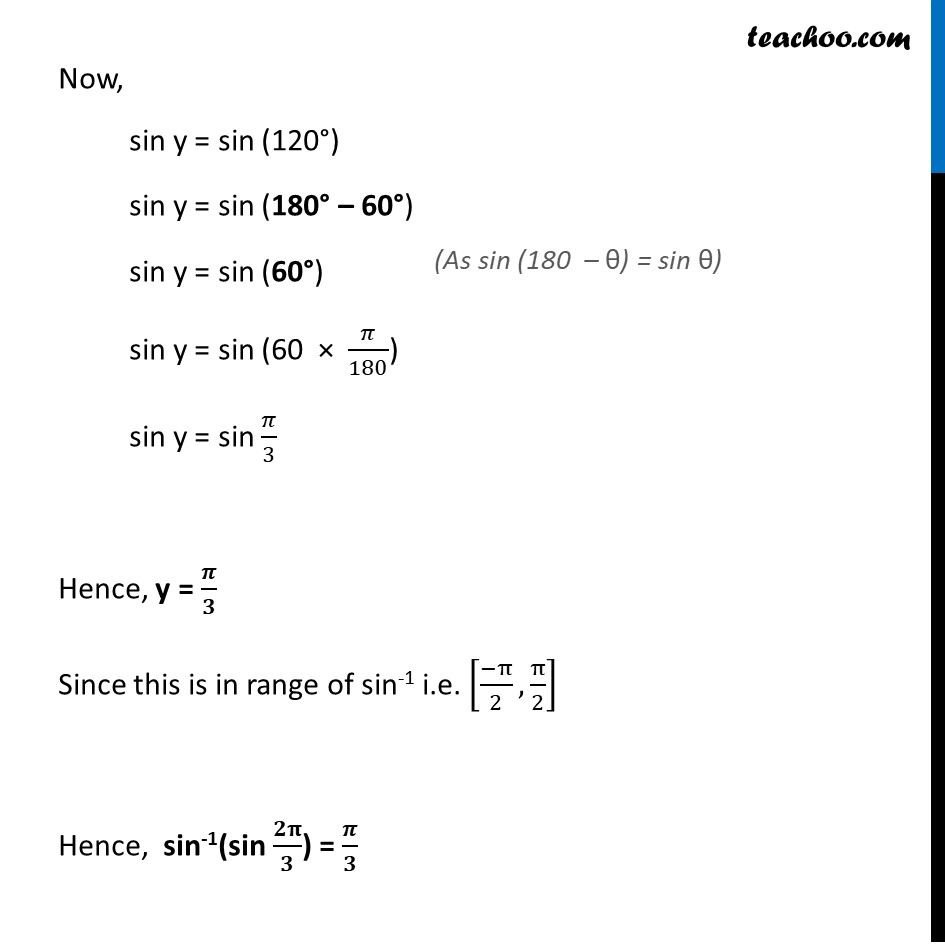Ex 2.2

Chapter 2 Class 12 Inverse Trigonometric Functions
Serial order wiseLearn in your speed, with individual attention - Teachoo Maths 1-on-1 Class

### Transcript

Ex 2.2, 10 Find the values of sin-1(sin⁡〖2π/3〗 ) Let y = sin-1 (sin 2𝜋/3) sin y = sin 2𝜋/3 sin y = sin (120°) But, Range of sin-1 is [(−π)/2, π/2] i.e. [-90° ,90° ] Hence, y = 120° not possible Now, sin y = sin (120°) sin y = sin (180° – 60°) sin y = sin (60°) sin y = sin (60 × 𝜋/180) sin y = sin 𝜋/3 Hence, y = 𝝅/𝟑 Since this is in range of sin-1 i.e. [(−π)/2, π/2] Hence, sin-1(sin 𝟐𝛑/𝟑) = 𝝅/𝟑